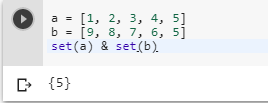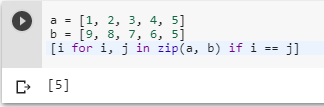2 views
in Python

I want to take two lists and find the values that appear in both.

a = [1, 2, 3, 4, 5]

b = [9, 8, 7, 6, 5]

returnMatches(a, b)

would return , for instance.

by (106k points)

There are many ways to compare two lists in python and return their matches some of the important methods are as follows:-

a = [1, 2, 3, 4, 5]

b = [9, 8, 7, 6, 5]

set(a) & set(b)Another method you can use is the list comprehensions if an order is significant, below is the code that shows how we use list-comprehension:-

a = [1, 2, 3, 4, 5]

b = [9, 8, 7, 6, 5]

[i for i, j in zip(a, b) if i == j]